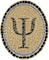Psellos
Life So Short, the Craft So Long to Learn

# Further OCaml GC Disharmony

January 25, 2015

While working on an OCaml app to run in the iPhone Simulator, I discovered another wrapper code construct that looks plausible but is incorrect. I was able to reproduce the error in a small example under OS X, so I am writing it up for the record.

The error is in code that calls from Objective C into OCaml. In an OCaml iOS app these calls happen all the time, since events originate in iOS. You can imagine events being formed originally from an Objective C-like substance, then being remanufactured into an OCaml material and passed through the interface.

As a teensy example, assume that you want to create a point and a rectangle in C and pass them to a function in OCaml. To make it interesting, assume that you want to count the fraction of time a rectangle with randomly chosen origin and size (uniform values in [0, 1]) contains the point (0.5, 0.5).

Here is some C code that does this (r4b.c):

``````#include <stdio.h>
#include <stdlib.h>
#include <limits.h>

#define CAML_NAME_SPACE
#include "caml/memory.h"
#include "caml/alloc.h"
#include "caml/callback.h"

double dran()
{
static unsigned long state = 72;
state = state * 6364136223846793005L + 1442695040888963407L;
return (double) state / (double) ULONG_MAX;
}

static value Val_point(double x, double y)
{
CAMLparam0();
CAMLlocal3(point, fx, fy);
point = caml_alloc(2, 0);
fx = caml_copy_double(x);
fy = caml_copy_double(y);
Store_field(point, 0, fx);
Store_field(point, 1, fy);
CAMLreturn(point);
}

static value ran_rect()
{
CAMLparam0();
CAMLlocal5(rect, fx, fy, fwd, fht);
rect = caml_alloc(4, 0);
fx = caml_copy_double(dran());
fy = caml_copy_double(dran());
fwd = caml_copy_double(dran());
fht = caml_copy_double(dran());
Store_field(rect, 0, fx);
Store_field(rect, 1, fy);
Store_field(rect, 2, fwd);
Store_field(rect, 3, fht);
CAMLreturn(rect);
}

int main(int ac, char *av[])
{
CAMLparam0();
int ct, i;
CAMLlocal2(point, isinside);
value *inside;

caml_main(av);

point = Val_point(0.5, 0.5);
inside = caml_named_value("inside");

ct = 0;
for (i = 0; i < 1000000000; i++) {
isinside = caml_callback2(*inside, point, ran_rect());
if (Bool_val(isinside))
ct++;
}
printf("%d (%f)\n", ct, (double) ct / (double) 1000000000);
CAMLreturnT(int, 0);
}``````

This OCaml code tests whether the point is inside the rectangle (inside.ml):

``````let inside (px, py) (x, y, w, h) =
px >= x && px <= x +. w && py >= y && py <= y +. h

let () = Callback.register "inside" inside``````

The basic idea is sound, but if you build and run this code in OS X you see the following:

``````\$ ocamlopt -output-obj -o inside.o inside.ml
\$ W=`ocamlopt -where`; clang -I \$W -L \$W -o r4b r4b.c inside.o -lasmrun
\$ r4b
Segmentation fault: 11``````

You, reader, are probably way ahead of me as usual, but the problem is in this line:

``isinside = caml_callback2(*inside, point, ran_rect());``

The problem is that `ran_rect()` allocates OCaml memory to hold the rectangle and its float values. Every once in a while, this will cause a garbage collection. If the OCaml value for `point` has already been calculated and saved aside (i.e., if the parameters to `caml_callback2` are evaluated left to right), this can cause the calculated value to become invalid before the call happens. This will lead to trouble: either a crash (as here) or, worse, the wrong answer.

The solution is to call `ran_rect()` beforehand:

``````int main(int ac, char *av[])
{
CAMLparam0();
int ct, i;
CAMLlocal3(point, rect, isinside);
value *inside;

caml_main(av);

point = Val_point(0.5, 0.5);
inside = caml_named_value("inside");

ct = 0;
for (i = 0; i < 1000000000; i++) {
rect = ran_rect();
isinside = caml_callback2(*inside, point, rect);
if (Bool_val(isinside))
ct++;
}
printf("%d (%f)\n", ct, (double) ct / (double) 1000000000);
CAMLreturnT(int, 0);
}``````

This revised version works correctly:

``````\$ ocamlopt -output-obj -o inside.o inside.ml
\$ W=`ocamlopt -where`; clang -I \$W -L \$W -o r4b r4b.c inside.o -lasmrun
\$ r4b
140625030 (0.140625)``````

(If my calculations are correct, the expected fraction is indeed 9/64, or 0.140625.)

In retrospect the problem is obvious, but I’ve wondered for years whether this construct is OK. As far as I can tell it isn’t explicitly forbidden by any of the GC Harmony Rules. In many ways, though, it’s related to Rule 4: the calculated value to be passed is like a global value, in that it’s outside the reach of the `CAMLlocal()` macros.

A good rule of thumb seems to be that you shouldn’t write an expression as an argument to a function if it can cause OCaml allocation. If necessary, evaluate the expression before the call.

I hope this may help some other humble OCaml developer seeking to attune his or her life with the Garbage Collector. If you have any comments or encouragement, leave them below or email me at jeffsco@psellos.com.

Posted by: Jeffrey